• 沃尔什-哈达玛变换（Walsh-Hadamard Transform,WHT），是一种典型的非正弦函数变换，采用正交直角函数作为基函数，具有与傅里叶函数类似的性质，图像数据越是均匀分布，经过沃尔什-哈达玛变换后的数据越是集中于矩阵...
沃尔什-哈达玛变换（Walsh-Hadamard Transform,WHT），是一种典型的非正弦函数变换，采用正交直角函数作为基函数，具有与傅里叶函数类似的性质，图像数据越是均匀分布，经过沃尔什-哈达玛变换后的数据越是集中于矩阵的边角上，因此沃尔什变换具有能量集中的性质，可以用于压缩图像信息。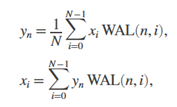在Matlab里计算如下：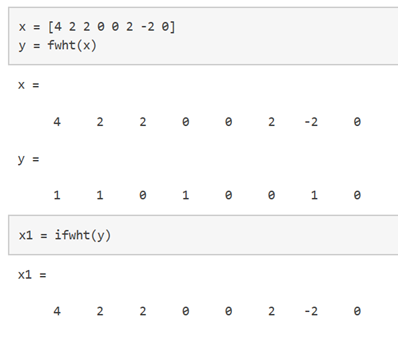采用sympy库（可以使用pip install sympy安装）计算如下：

#python 3.7.4,opencv4.1
#蔡军生 https://blog.csdn.net/caimouse/article/details/51749579
#
import numpy as np
import cv2
from matplotlib import pyplot as plt
from sympy import fwht, ifwht

#
x = [4, 2, 2, 0, 0, 2, -2, 0]
y = np.array(fwht(x))/len(x)
print(y)

z = np.array(ifwht(y))*len(y)
print(z)

#
cv2.waitKey(0)
cv2.destroyAllWindows()



结果输出如下：

[1 0 1 0 1 1 0 0]

[4 2 2 0 0 2 -2 0]

玩转人工智能库-深入浅出OpenCVhttps://edu.csdn.net/course/detail/26616

Python游戏开发入门

http://edu.csdn.net/course/detail/5690

你也能动手修改C编译器

http://edu.csdn.net/course/detail/5582

展开全文opencv
• 沃尔什-哈达玛变换（Walsh-Hadmard Transform,WHT），是一种典型的非正弦函数变换，采用正交直角函数作为基函数，具有与傅里叶函数类似的性质，图像数据越是均匀分布，经过沃尔什-哈达玛变换后的数据越是集中于矩阵...
沃尔什-哈达玛变换（Walsh-Hadmard Transform,WHT），是一种典型的非正弦函数变换，采用正交直角函数作为基函数，具有与傅里叶函数类似的性质，图像数据越是均匀分布，经过沃尔什-哈达玛变换后的数据越是集中于矩阵的边角上，因此沃尔什变换具有能量集中的性质，可以用于压缩图像信息。Matlab中的Hadamard函数：格式：H=hadamard( n ) ，返回一个 n * n的hadamard矩阵。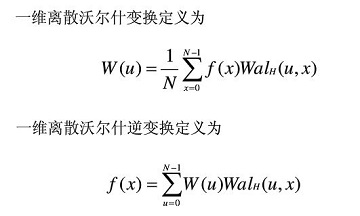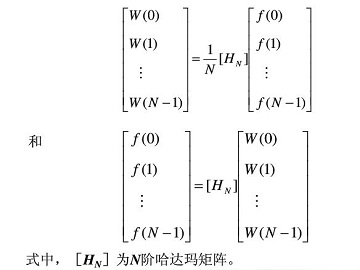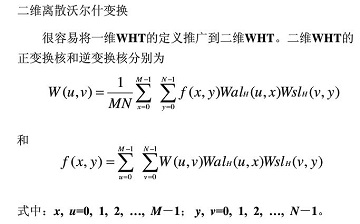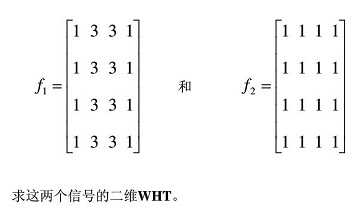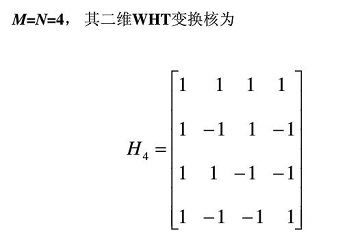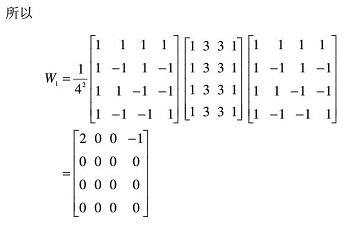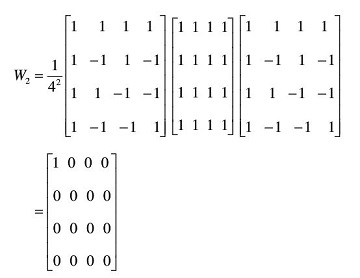下面对lena图像进行沃尔什-哈达玛变换与逆变换的Matlab实现：[plain] view plain copyclc;  clear all;  im_l=imread('C:\Users\DELL\Desktop\lena.jpg');  im_l1=im2double(im_l);  im_l2=rgb2gray(im_l1);    %对图像进行哈达玛变换  H=hadamard(512);%产生512X512的Hadamard矩阵  haImg=H*im_l2*H;  haImg2=haImg/512;    %对图像进行哈达玛逆变换  hhaImg=H'*haImg2*H';  hhaImg2=hhaImg/512;    haImg1=im2uint8(haImg);  hhaImg1=im2uint8(hhaImg2);    subplot(2,2,1);  imshow(im_l);  title('原图');    subplot(2,2,2);  imshow(im_l2);  title('灰度图');    subplot(2,2,3);  imshow(haImg2);  title('图像的二维离散Hadamard变换');    subplot(2,2,4);  imshow(hhaImg1);  title('图像的二维离散Hadamard逆变换');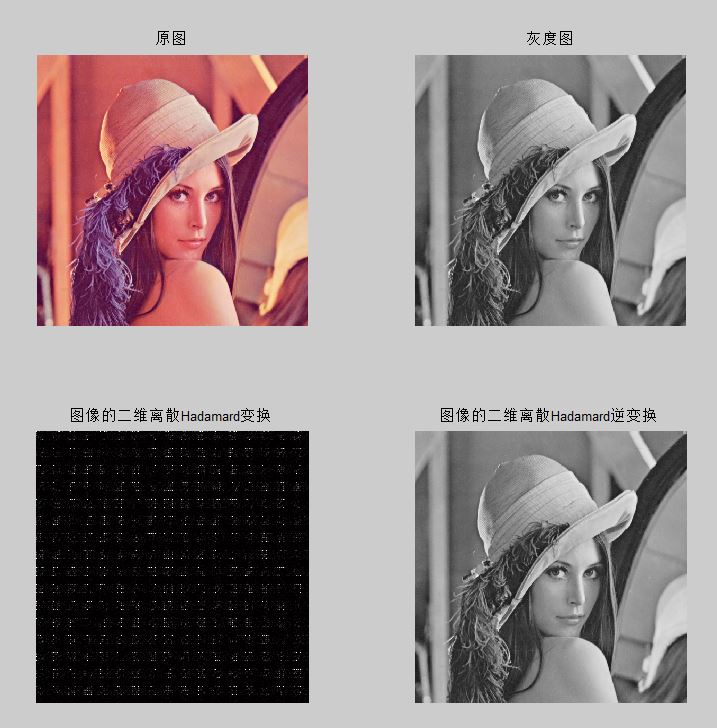转自https://blog.csdn.net/geekmanong/article/details/50038611
展开全文压缩感知
• 小波变换分解重构(Matlab)基本的matlab图像处理函数的使用代码块运行效果傅里叶变换(DFT)对图像进行傅里叶正变换去除部分高频分量后...变换(WHT)对图像进行WHT变换去除部分高频分量后对图像进行WHT变换小波分解重构...
文章目录数字图像处理-DFT&DCT&WHT&小波变换分解重构(Matlab)基本的matlab图像处理函数的使用代码块运行效果傅里叶变换(DFT)对图像进行傅里叶正变换去除部分高频分量后对图像进行傅里叶逆变换离散余弦变换(DCT)对图像进行DCT正变换去除部分高频分量后对图像进行DCT逆变换沃尔什哈达玛变换(WHT)对图像进行WHT正变换去除部分高频分量后对图像进行WHT逆变换小波分解重构一级小波分解一级小波重构二级小波分解二级小波重构
数字图像处理-DFT&DCT&WHT&小波变换分解重构(Matlab)
基本的matlab图像处理函数的使用

函数
功能

imread('图像名')
读取图像

imshow(color_pic)
显示图像

rgb2gray(color_pic)
将彩色图像转换成灰度图像

imhist(gray_pic,n)
查看灰度图像的灰度直方图

代码块
%-----------------Matlab基本图像处理函数使用------------------
clear ;
close all;
color_pic=imread('lena512color.bmp');  %读取图像
figure('name','图像读取');
subplot(2,2,1);
imshow(color_pic);   %显示图像
title('原彩色图像');
gray_pic=rgb2gray(color_pic);  %将彩色图转换成灰度图
subplot(2,2,2);
imshow(gray_pic);
title('灰度图像');
subplot(2,2,3);
imhist(gray_pic);   %查看灰度直方图，默认n=256,256个长度为1的灰度空间
title('灰度直方图256等级');
subplot(2,2,4);
imhist(gray_pic,64);%n=64，64个长度为4的灰度空间
title('灰度直方图64等级');

运行效果傅里叶变换(DFT)
对图像进行傅里叶正变换
%------------------傅里叶变换------------------
clear;  %清除变量
close all; %关闭生成的画图窗口
color_pic=imread('lena512color.bmp');  %读取图像
gray_pic=rgb2gray(color_pic);  %将彩色图转换成灰度图
figure('name','傅里叶变换'); %傅里叶变换
subplot(2,2,1);
imshow(gray_pic);
title('原灰度图像');
Fourier=fft2(gray_pic);  %对灰度图像进行傅里叶正变换
log_Fourier=log(abs(Fourier)+1); %取模并进行缩放，调高频谱图像的低灰度值而对高灰度值仅可能减小
subplot(2,2,2);
imshow(log_Fourier,[]);    %未进行频谱搬移时的频谱图
title('傅里叶变换频谱图');
Fourier_shift=fftshift(Fourier); %将频谱图中零频率成分移动至频谱图中心
log_Fourier_shift=log(abs(Fourier_shift)+1); %取模并进行缩放，对于（0，1）之间的x值经过取对数后会变成负值，而log（x+1）则将所有的x值映射到正数范围内
subplot(2,2,3);
imshow(log_Fourier_shift,[]);
title('频移后的频谱图');


程序运行效果：总结：
在图像的傅里叶频谱中，原空间域图像上的灰度突变部位、图像结构复杂的区域、图像细节及干扰噪声等信息集中在高频区，原空间域图像上灰度变化平缓部位的信息(图像轮廓)集中在低频区。
低频部分(图像轮廓)对应于未进行频移的傅里叶频谱的4个边角角部分，由于低频部分能量较集中，因而在频谱图上的视觉效果较亮。当进行频移后，低频部分移至频谱中央，中央处最亮。

去除部分高频分量后对图像进行傅里叶逆变换
%-----------------设置阈值滤除高频  傅里叶逆变换----------------
clear;
close all;
color_pic=imread('lena512color.bmp');  %读取图像
gray_pic=rgb2gray(color_pic);  %将彩色图转换成灰度图
threshold=[100000,30000,5000,500];  %设置不同阈值 (高频部分能量低)
figure('name','傅里叶逆变换图像');
for i=1:4
Fourier=fft2(gray_pic);  %对灰度图像进行傅里叶正变换
Fourier_shift=fftshift(Fourier); %将频谱图中零频率成分移动至频谱图中心
h_Fourier_shift=abs(Fourier_shift);% 取傅里叶变换后幅度模值，使灰度值为正数
Fourier_shift(h_Fourier_shift<threshold(i))=0;  %取阈值消除部分高频
IFourier=real(ifft2(ifftshift(Fourier_shift)));  %傅里叶逆变换，要记得先把频移的频谱频移回去
ret=uint8(IFourier); %将灰度级映射到0-255上
subplot(2,2,i);
imshow(ret);
str=num2str(threshold(i));
title(['阈值:',str]);
end


程序运行效果：总结：
因为高频部分能量较低，即傅里叶变换后的高频部分幅度值较低，当设置的阈值越小时，保留了更多低频部分，即轮廓部分保留下来，图像也就恢复的越接近原图。

离散余弦变换(DCT)
对图像进行DCT正变换
%----------------DCT变换------------------
clear;
close all;
color_pic=imread('lena512color.bmp');  %读取图像
gray_pic=rgb2gray(color_pic);  %将彩色图转换成灰度图
figure('name','DCT变换');
DCT=dct2(gray_pic);  %DCT变换
h_DCT=abs(DCT);  %DCT变换后的幅度
log_DCT=log(h_DCT);   %取模并进行缩放，调高频谱图像的低灰度值而对高灰度值仅可能减小
imshow(log_DCT,[]);
title('DCT变换');
colormap(gray(4));  %重新设置灰度级为4，便于查看DCT变换后的频谱图特点
colorbar;   %显示颜色栏


程序运行效果：总结：
从DCT频谱图易看出，低频部分(图像轮廓)能量集中在左上角，因此可进行图像压缩。

去除部分高频分量后对图像进行DCT逆变换
%----------------取阈值去除高频分量 DCT逆变换------------------
clear;
close all;
color_pic=imread('lena512color.bmp');  %读取图像
gray_pic=rgb2gray(color_pic);  %将彩色图转换成灰度图
threshold=[200,100,10,1];  %设置不同阈值 (高频部分能量低)
for i=1:4
DCT=dct2(gray_pic); %DCT正变换
h_DCT=abs(DCT);  %DCT变换后的幅度
DCT(h_DCT<threshold(i))=0;  %DCT变换后取模得出的幅度值若小于20则至0
IDCT=uint8(idct2(DCT));  %对图像进行DCT逆变换，并将灰度级映射到0-255上
subplot(2,2,i);
imshow(IDCT);
str=num2str(threshold(i));
title(['阈值:',str]);
end


程序运行效果：总结：
因为高频部分能量较低，即DCT变换后的高频部分幅度值较低，当设置的阈值越小时，保留了更多低频部分，即轮廓部分保留下来，图像也就恢复的越接近原图。

沃尔什哈达玛变换(WHT)
对图像进行WHT正变换
%------------------沃尔什哈达玛变换------------------
clear;
close all;
color_pic=imread('lena512color.bmp');  %读取图像
gray_pic=rgb2gray(color_pic);  %将彩色图转换成灰度图
figure('name','沃尔什哈达玛');
subplot(2,2,1);
imshow(gray_pic);
title('原灰度图像');    %imread读入源图像为三维，所以不能进行D=A*X*A
im2double_gray_pic=im2double(gray_pic);%必须对读取的图像做I=im2double(I),函数im2double 将其值归一化到0～1之间
Hada=hadamard(512); %生成512x512哈达玛矩阵
Hada_pic=Hada*(im2double_gray_pic)*Hada;
Hada_pic2=Hada_pic/512;  %沃尔什哈达玛变换记得/(N*N=512*512)，此处只除512是为了频谱图效果好看
subplot(2,2,2);
imshow(Hada_pic2);
title('沃尔什哈达玛变换');


程序运行效果：去除部分高频分量后对图像进行WHT逆变换
%----------------取阈值去除高频分量 沃尔什哈达玛逆变换------------------
clear;
close all;
color_pic=imread('lena512color.bmp');  %读取图像
gray_pic=rgb2gray(color_pic);  %将彩色图转换成灰度图
im2double_gray_pic=im2double(gray_pic);%必须对读取的图像做I=im2double(I),函数im2double 将其值归一化到0～1之间
Hada=hadamard(512);%生成512x512哈达玛矩阵
Hada_pic=Hada*(im2double_gray_pic)*Hada;
threshold=[0.5,0.3,0.2,0.1];  %设置不同阈值 (高频部分能量低)
for i=1:4
Hada_pic2=Hada_pic/(512);  %沃尔什哈达玛变换记得/(N*N=512*512)，此处只除512是为了频谱图效果好看
h_Hada=abs(Hada_pic2);
Hada_pic2(h_Hada<threshold(i))=0; %取阈值消除部分高频
IHada_pic=Hada'*Hada_pic2*Hada';% Hada'是Hada的转置矩阵
IHada_pic2=im2uint8(IHada_pic/512); %将灰度级转换为255级，否则灰度值大于255，图像太亮，呈现一片白
subplot(2,2,i);
imshow(IHada_pic2);
str=num2str(threshold(i));
title(['阈值:',str]);
end;


程序运行效果：总结：
因为高频部分能量较低，即WHT变换后的高频部分幅度值较低，当设置的阈值越小时，保留了更多低频部分，即轮廓部分保留下来，图像也就恢复的越接近原图。

小波分解重构
一级小波分解
%-------------------小波变换一级分解，小波基函数选db4-----------------------
clear;
close all;
color_pic=imread('lena512color.bmp');  %读取图像
gray_pic=rgb2gray(color_pic);  %将彩色图转换成灰度图
figure('name','小波变换一级分解');
[c,s]=wavedec2(gray_pic,1,'db4'); %小波一级分解,小波基函数采用db4
ca1=appcoef2(c,s,'db4',1);   %低频分量
ch1=detcoef2('h',c,s,1);     %高频水平分量
cv1=detcoef2('v',c,s,1);     %高频垂直分量
cd1=detcoef2('d',c,s,1);     %高频对角分量
subplot(2,2,1);imshow(ca1,[]);title('LL1');
subplot(2,2,2);imshow(ch1,[]);title('HL1');
subplot(2,2,3);imshow(cv1,[]);title('LH1');
subplot(2,2,4);imshow(cd1,[]);title('HH1');


程序运行效果：一级小波重构
%-------------------小波变换一级重构，小波基函数选db4-----------------------
clear;
close all;
color_pic=imread('lena512color.bmp');  %读取图像
gray_pic=rgb2gray(color_pic);  %将彩色图转换成灰度图
figure('name','小波变换一级重构');
[c,s]=wavedec2(gray_pic,1,'db4'); %小波一级分解,小波基函数采用db4
re_ca1=wrcoef2('a',c,s,'db4',1); %重建第一层低频分量系数
re_ch1=wrcoef2('h',c,s,'db4',1); %重建第一层高频水平分量系数
re_cv1=wrcoef2('v',c,s,'db4',1); %重建第一层高频垂直分量系数
re_cd1=wrcoef2('d',c,s,'db4',1); %重建第一层高频对角分量系数
re_set1=[re_ca1,re_ch1;re_cv1,re_cd1];  %将各个分量图像拼接在一张图像
subplot(1,2,1);imshow(re_set1,[]);title('第一层小波系数的重构');
re_img1=re_ca1+re_ch1+re_cv1+re_cd1;%将各个分量合并复原
subplot(1,2,2);imshow(re_img1,[]);title('一级重构图像');


程序运行效果：二级小波分解
%-------------------小波变换二级分解，小波基函数选db4)-----------------------
clear;
close all;
color_pic=imread('lena512color.bmp');  %读取图像
gray_pic=rgb2gray(color_pic);  %将彩色图转换成灰度图
figure('name','小波变换二级分解');
[c,s]=wavedec2(gray_pic,2,'db4'); %小波二级分解
%小波一级分解分量
ca1=appcoef2(c,s,'db4',1);   %低频分量
ch1=detcoef2('h',c,s,1);     %高频水平分量
cv1=detcoef2('v',c,s,1);     %高频垂直分量
cd1=detcoef2('d',c,s,1);     %高频对角分量
%显示第1级分解各分量
subplot(4,4,[3,4,7,8]);imshow(ch1,[]);title('HL1');
subplot(4,4,[9,10,13,14]);imshow(cv1,[]);title('LH1');
subplot(4,4,[11,12,15,16]);imshow(cd1,[]);title('HH1');
%提取第2层的各分量
ca2=appcoef2(c,s,'db4',2);   %低频分量
ch2=detcoef2('h',c,s,2);     %高频水平分量
cv2=detcoef2('v',c,s,2);     %高频垂直分量
cd2=detcoef2('d',c,s,2);     %高频对角分量
%显示第2级分解各分量
subplot(4,4,1);imshow(ca2,[]);title('LL2');
subplot(4,4,2);imshow(ch2,[]);title('HL2');
subplot(4,4,5);imshow(cv2,[]);title('LH2');
subplot(4,4,6);imshow(cd2,[]);title('HH2');


程序运行效果：二级小波重构
%-------------------小波变换2级重构，小波基函数选db4-----------------------
clear;
close all;
color_pic=imread('lena512color.bmp');  %读取图像
gray_pic=rgb2gray(color_pic);  %将彩色图转换成灰度图
figure('name','小波变换二级重构');
[c,s]=wavedec2(gray_pic,2,'db4'); %小波二级分解
re_ca2=wrcoef2('a',c,s,'db4',2);  %重建第二层低频分量系数
re_ch2=wrcoef2('h',c,s,'db4',2);  %重建第二层高频水平分量系数
re_cv2=wrcoef2('v',c,s,'db4',2);  %重建第二层高频垂直分量系数
re_cd2=wrcoef2('d',c,s,'db4',2);  %重建第二层高频对角分量系数
re_set2=[re_ca2,re_ch2;re_cv2,re_cd2]; %将各个分量图像拼接在一张图像
subplot(1,2,1);imshow(re_set2,[]);title('第二层小波系数的重构');
re_img2=re_ca2+re_ch2+re_cv2+re_cd2; %将各个分量合并复原
subplot(1,2,2);imshow(re_img2,[]);title('二级重构图像');


程序运行效果：展开全文matlab idft 小波分析
• 该算法通过次优PTS算法对OFDM输入信号进行WHT变换，降低了信号的自相关性，提升了算法抑制PAPR的性能。仿真结果表明，相比次优PTS算法，联合算法在相同分组数情况下，PAPR性能提升了0.6 dB。在相近PAPR性能条件下，...
• 沃尔什-哈达玛变换（Walsh-Hadmard Transform,WHT） 沃尔什-哈达玛变换（Walsh-Hadmard Transform,WHT） 1. 前言 2.数学表达式 2.1 一维哈达玛正变换及反变换 2.2 二维哈达玛正变换及反变换 2.3 沃尔什变换 2.4 ...
沃尔什-哈达玛变换（Walsh-Hadmard Transform,WHT）

沃尔什-哈达玛变换（Walsh-Hadmard Transform,WHT）

1. 前言
2.数学表达式

2.1 一维哈达玛正变换及反变换
2.2 二维哈达玛正变换及反变换
2.3 沃尔什变换
2.4 沃尔什离散变换

3. 代码实现

1. 前言
哈达玛变换是利用哈达玛矩阵作为变换矩阵新实施的遥感多光谱域变换。哈达玛矩阵为一个对称的正交矩阵。哈达变换实际是将坐标轴旋转45°的正交变换。
沃尔什-哈达玛变换（Walsh-Hadmard Transform,WHT），是一种典型的非正弦函数变换，采用正交直角函数作为基函数，具有与傅里叶函数类似的性质，图像数据越是均匀分布，经过沃尔什-哈达玛变换后的数据越是集中于矩阵的边角上，因此沃尔什变换具有能量集中的性质，可以用于压缩图像信息。
2.数学表达式

哈达玛矩阵：哈达玛矩阵的元素由+1和-1组成的下交方阵
哈达玛变换要求图像的大小为$N=2^n$

2.1 一维哈达玛正变换及反变换
正换公式如下：
$H(u) = \frac{1}{n}\sum_{x=0}^{n-1}{f(x)(-1)^{\sum_{i=0}^{n-1}{b_i(x)b_i(u)}}}$
反变换公式如下：
$f(x) = \sum_{u=0}^{n-1}{H(u)(-1)^{\sum_{i=0}^{n-1}{b_i(x)b_i(u)}}}$
2.2 二维哈达玛正变换及反变换
正换公式如下：
$H(u,v) = \frac{1}{N}\sum_{x=0}^{N-1}\sum_{y=0}^{N-1}{f(x,y)(-1)^{\sum_{i=0}^{n-1}{[b_i(x)b_i(u)+b_i(y)b_i(v)]}}}$
反变换公式如下：
$f(x,y) = \frac{1}{N}\sum_{u=0}^{N-1}\sum_{v=0}^{N-1}{H(u,v)(-1)^{\sum_{i=0}^{n-1}{[b_i(x)b_i(u)+b_i(y)b_i(v)]}}}$
2.3 沃尔什变换
由哈达玛的表达式可以知道，哈达玛变换矩阵的列率的排列是无序的，如果将哈达玛核进行列率的排序，即得到的有序的变换就是沃尔什(Walsh)变换。
一维Walsh变换核为：
$g(x,u) = \frac{1}{N}\prod_{i=0}^{N-1}{(-1)^{\sum_{i=0}^{n-1}b_i(x)b_{n-1-i}(u)}}$
二维沃尔什正变换
$W(u,v) = \frac{1}{N}\sum_{x=0}^{N-1}\sum_{y=0}^{N-1}{f(x,y)\prod_{i=0}^{n-1}(-1)^{\sum_{i=0}^{n-1}{[b_i(x)b_{n-1-i}(u)+b_i(y)b_{n-1-i}(v)]}}}$
二维沃尔什反变换
$f(x,y) = \frac{1}{N}\sum_{x=0}^{N-1}\sum_{y=0}^{N-1}{W(u,v)\prod_{i=0}^{n-1}(-1)^{\sum_{i=0}^{n-1}{[b_i(x)b_{n-1-i}(u)+b_i(y)b_{n-1-i}(v)]}}}$
2.4 沃尔什离散变换
一维离散沃尔什正变换
$W(u) = \frac{1}{N}\sum_{x=0}^{N-1}{f(x)Wal_H(u,x)}$
一维离散沃尔什反变换
$f(x) = \frac{1}{N}\sum_{x=0}^{N-1}{W(u)Wal_H(u,x)}$
二维离散沃尔什正变换
$W(u,v) = \frac{1}{MN}\sum_{x=0}^{M-1}\sum_{y=0}^{N-1}{f(x,y)Wal_H(u,v)Wsl_H(v,y)}$
二维离散沃尔什反变换
$f(x,y) = \frac{1}{MN}\sum_{x=0}^{M-1}\sum_{y=0}^{N-1}{W(u,v)Wal_H(u,v)Wsl_H(v,y)}$
其中：$x,u=0,1,2,...,M-1;y,v=0,1,2,...N-1$
3. 代码实现

import scipy
from scipy.linalg import hadamard
import cv2
import numpy as np

# 注意图像大小一定是2的幂次方
src = cv2.imread('resources/images/lena.png',0)
srcf = np.float32(src)
h1,h2 = src.shape

# 生成哈达玛变换矩阵
H1 = hadamard(h1)
H2 = hadamard(h2)

# 哈达玛变换
HJ = H1.dot(srcf).dot(H2)
J = HJ / np.sqrt(h1*h2)

# 哈达玛变换逆变换
RJ = H1.T.dot(J).dot(H2.T)
HRJ = RJ / np.sqrt(h1*h2)

J = np.clip(J,0,255).astype(np.uint8)
HRJ = np.clip(HRJ,0,255).astype(np.uint8)

cv2.imshow(u'Source',src)
cv2.imshow(u'Hadamard Transform Result',J)
cv2.imshow(u'hadamard Transform Inverse Result',HRJ)
cv2.waitKey()
cv2.destroyAllWindows()

程序运行结果：第一张图像为原图像，第二张为哈达玛变换结棍，第三张为哈达玛反变换结果


展开全文计算机视觉 人工智能 图像处理
• 函数 hough.m 基于时频工具箱中的函数 htl.m 但坐标原点不同。... 时间 t（霍夫变换中的 y）介于 0 和 1 之间，因此 theta 约为 90，rho 约为 0。我将不胜感激任何建议和问题！ 他们会对我很有帮助。 谢谢！matlab
• matlab时频分析工具箱-WHT.zip 本帖最后由 大菜鸟 于 2014-7-24 09:28 编辑 最近做直流输电故障极波的信号处理，翻来覆去找不到各种变换的matlab程序，不过现在项目做得差不多了我就把这些东西都贴上来共享一下...matlab
• 图像时频变换DCT FFT DWT WHT的C#源码
• WHT变换：非正弦函数变换，采用正交直角函数作为基函数； 离散余弦变换： 小波变换：多分辨率分析，渐进传输；3、预测编码预测编码，通过输入的值与预测器的值的偏差进行编码，这样，原数据就可以压缩成一个初始值...编码 压缩
• 在本文中，我们设计了一种新的迭代低复杂度算法，用于使用K稀疏WHT计算N维信号的Walsh-Hadamard变换WHT）。 我们假设N是2的幂，并且K = O（N ^α），对于某个α∈（0,1），在N中亚线性缩放。 假设一个非零变换域...
• 为了解决Wigner-Hough变换对多分量线性调频(LFM)信号特征提取受噪声困扰的问题，提出采用...通过对WHT，SPWD-Hough变换以及改进WHT 3种方法进行计算机仿真比较结果表明，改进的WHT对多分量LFM信号的特征提取效果最好．
• 采用哈达玛排列的沃尔什函数进行的变换称为沃尔什-哈达玛变换，简称WHT或直称哈达玛变换。 沃尔什函数 沃尔什函数有三种不同的函数定义，但都可由拉德梅克函数构成。 1、按沃尔什排列的沃尔什函数 ...
• 为了比较Wigner-Hough变换(WHT)和Radon-Ambiguity变换(RAT)之间的差别，利用平稳高斯随机过程各态遍历特性，推导了RAT在采样点数较多的情况下，采样点数与输入、输出信噪比之间的关系表达式．从变换的实现方法、运算...
• 借助Wigner-Hough变换(WHT)可对目标多普勒参数进行较为精确的估计,但该方法由于计算量和存储量庞大而难以得到应用,需要进行改进。从运动目标回波特性出发,提出了“参数引导+局部搜索”的快速估计理念,并提出了实现该...
• 沃尔什-哈达玛变换（Walsh-Hadmard Transform,WHT），是一种典型的非正弦函数变换，采用正交直角函数作为基函数，具有与傅里叶函数类似的性质，图像数据越是均匀分布，经过沃尔什-哈达玛变换后的数据越是集中于矩阵...
• 沃尔什-哈达玛变换（Walsh-Hadmard Transform,WHT），是一种典型的非正弦函数变换，采用正交直角函数作为基函数，具有与傅里叶函数类似的性质，图像数据越是均匀分布，经过沃尔什-哈达玛变换后的数据越是集中于矩阵...